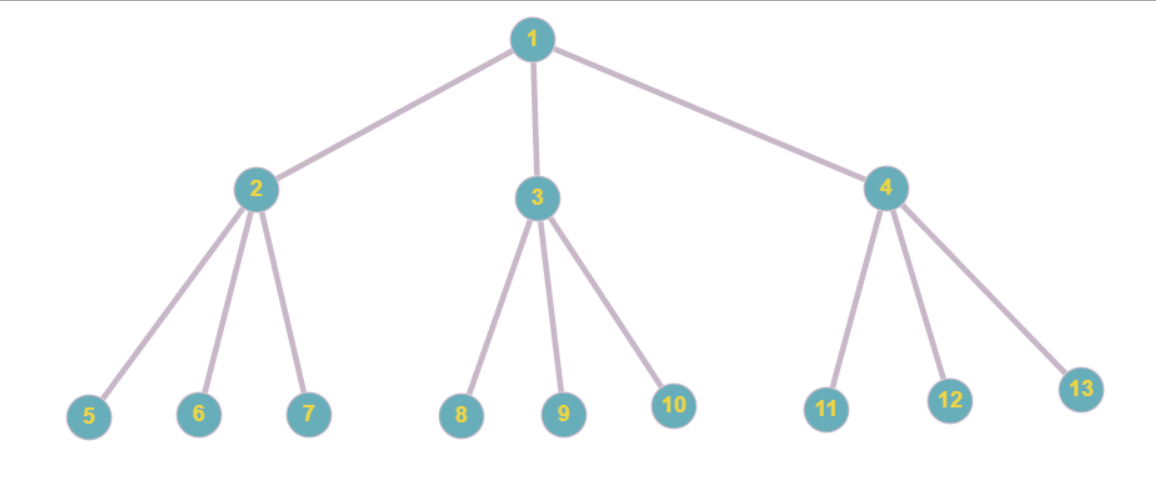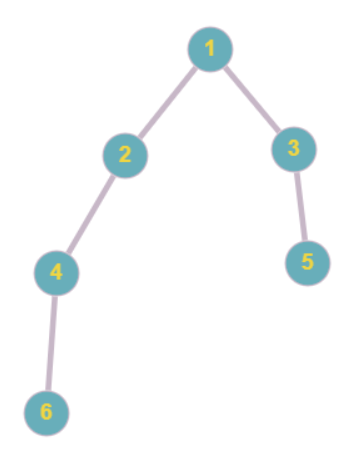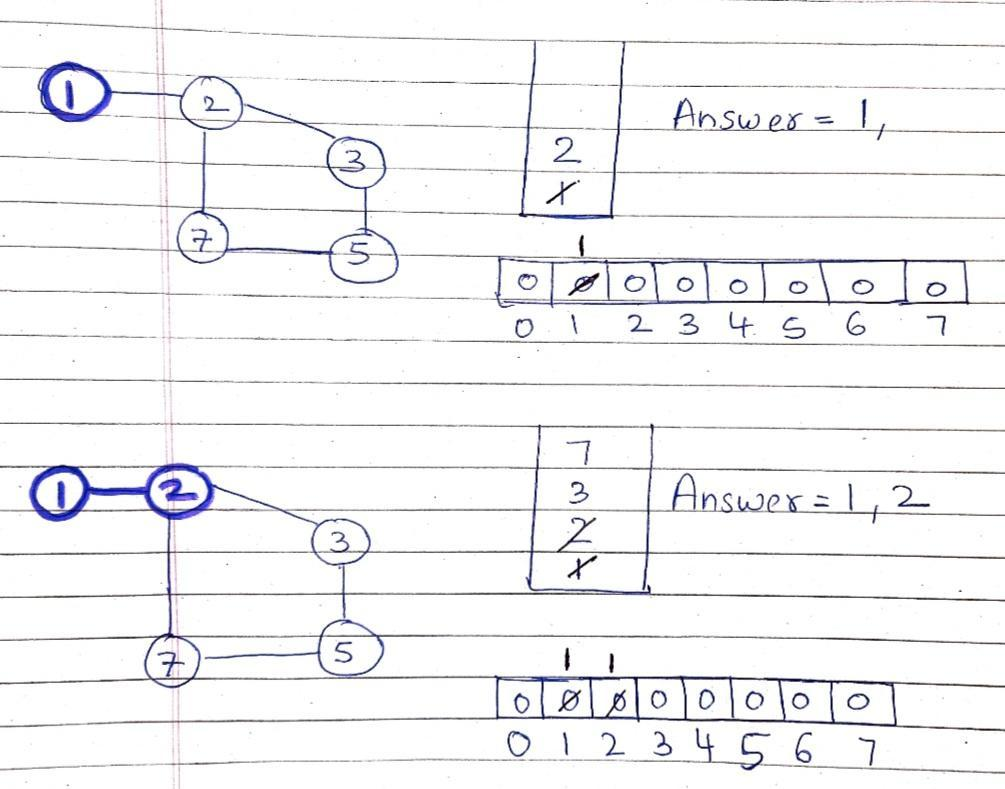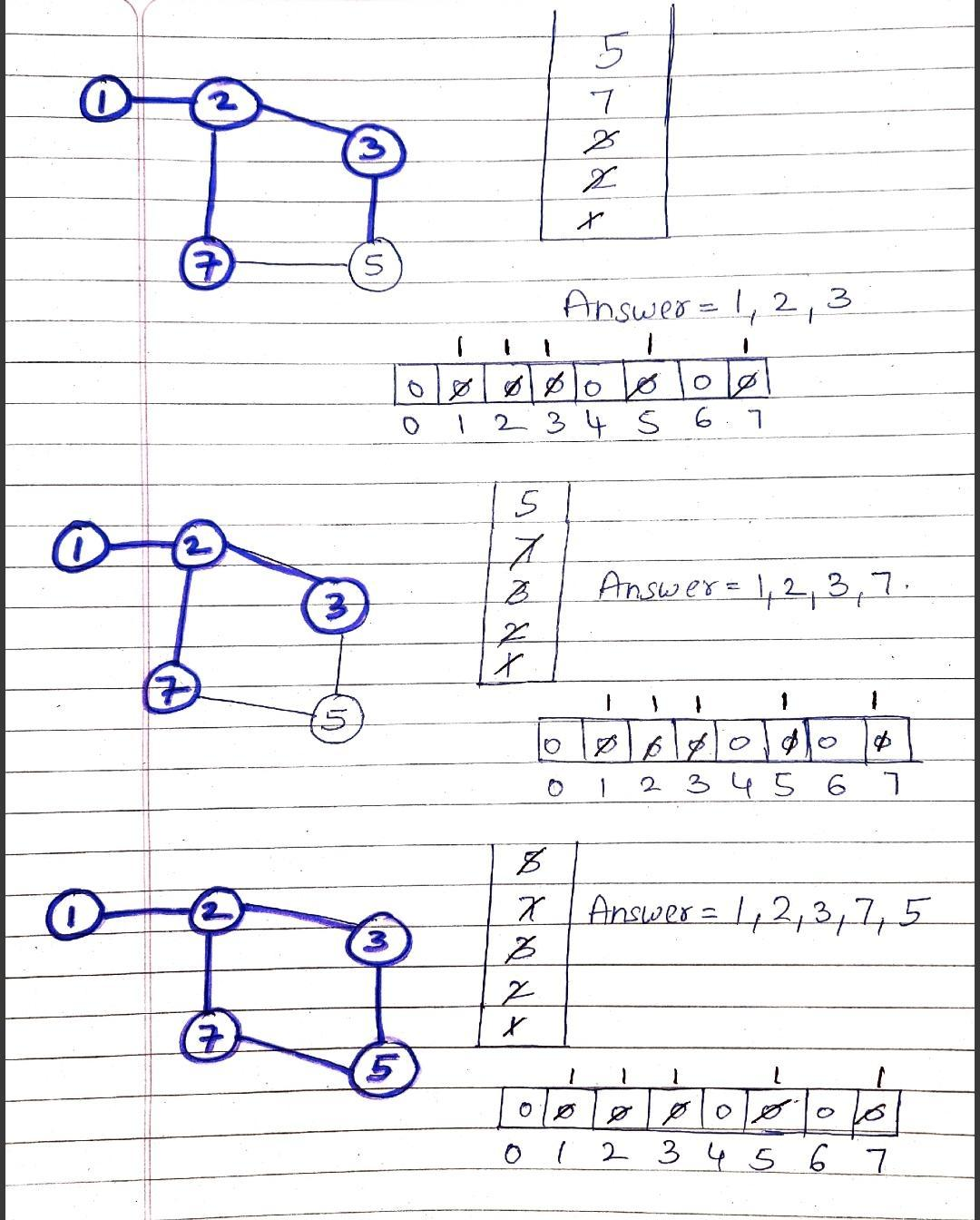# Breadth-First Search(BFS) : Level Order Traversal

Problem Statement: An Undirected Graph will be given. Return a vector of all the nodes of the Graph by Breadth-First Search ( BFS ) Technique.

Pre-req. : Queue STL, Introduction to graphs

Examples:

```Example 1:

Input:Output: 1 , 2 , 3 , 4 , 5 , 6 , 7, 8 , 9 , 10, 11, 12, 13```
```Example 2:

Input:Output: 1 , 2 , 3 ,4 , 5 , 6```

### Solution

Disclaimer: Don’t jump directly to the solution, try it out yourself first.

Intuition :

-> We will capture a node. After capturing this node, we will put this node’s value into our answer vector and mark its visited value as true. Now, this node will be having some adjacent nodes connected to it.

-> We will push all the adjacent nodes which are NOT VISITED into the queue data structure.

If we encounter any VISITED adjacent nodes will not be considering them because we don’t want to consider them twice.

-> By this way, we are covering all the nodes of the component breadth-wise.

Approach :

-> We need to have this before proceeding :

1. Queue Data structure
2. Visited Array – An array with all values initialised with 0.

-> We will push the 1st node into the queue data structure and mark it as visited. After this, we will find its adjacent nodes. If we get some unvisited node, we will simply push this adjacent node into the queue data structure

-> Now since the work of the 1st node is done, we will pop out this node from the queue. Now, this process goes on until the queue is not empty.Code:

## C++ code

``````#include <bits/stdc++.h>

using namespace std;
class Solution {
public:
vector < int > bfsOfGraph(int V, vector < int > adj[]) {
vector < int > bfs;
vector < int > vis(V, 0);
queue < int > q;
q.push(0);
vis = 1;
while (!q.empty()) {
int node = q.front();
q.pop();
bfs.push_back(node);

for (auto it: adj[node]) {
if (!vis[it]) {
q.push(it);
vis[it] = 1;
}
}
}

return bfs;
}
};

void addEdge(vector < int > adj[], int u, int v) {
}

void printAns(vector < int > & ans) {
for (int i = 0; i < ans.size(); i++) {
cout << ans[i] << " ";
}
}
int main() {
vector < int > adj;

Solution obj;
vector < int > ans = obj.bfsOfGraph(5, adj);
printAns(ans);
cout << endl;

return 0;
}``````

Output:

0 1 2 3 4

Time Complexity : O(N+E)

N = Nodes , E = travelling through adjacent nodes

Space Complexity : O(N+E) + O(N) + O(N)

Space for adjacency list, visited array, queue data structure

## Java Code

``````import java.util.*;
class Solution {
public static ArrayList < Integer > bfsOfGraph(int V, ArrayList < ArrayList < Integer >> adj) {

ArrayList < Integer > bfs = new ArrayList < > ();
boolean vis[] = new boolean[V];
Queue < Integer > q = new LinkedList < > ();

vis = true;

while (!q.isEmpty()) {
Integer node = q.poll();

// Get all adjacent vertices of the dequeued vertex s
// If a adjacent has not been visited, then mark it
// visited and enqueue it
for (Integer it: adj.get(node)) {
if (vis[it] == false) {
vis[it] = true;
}
}
}

return bfs;

// Code here
}

static void printAns(ArrayList < Integer > ans) {
for (int i = 0; i < ans.size(); i++) {
System.out.print(ans.get(i) + " ");
}
}
public static void main(String args[]) {

ArrayList < ArrayList < Integer >> adj = new ArrayList < > ();
for (int i = 0; i < 5; i++) {
}

ArrayList < Integer > ans = bfsOfGraph(5, adj);
printAns(ans);

}
}``````

Output:

0 1 2 3 4

Time Complexity : O(N+E)

N = Nodes , E = travelling through adjacent nodes

Space Complexity : O(N+E) + O(N) + O(N)

Space for adjacency list, visited array, queue data structure

Special thanks to Shreyas Vishwakarma for contributing to this article on takeUforward. If you also wish to share your knowledge with the takeUforward fam, please check out this article